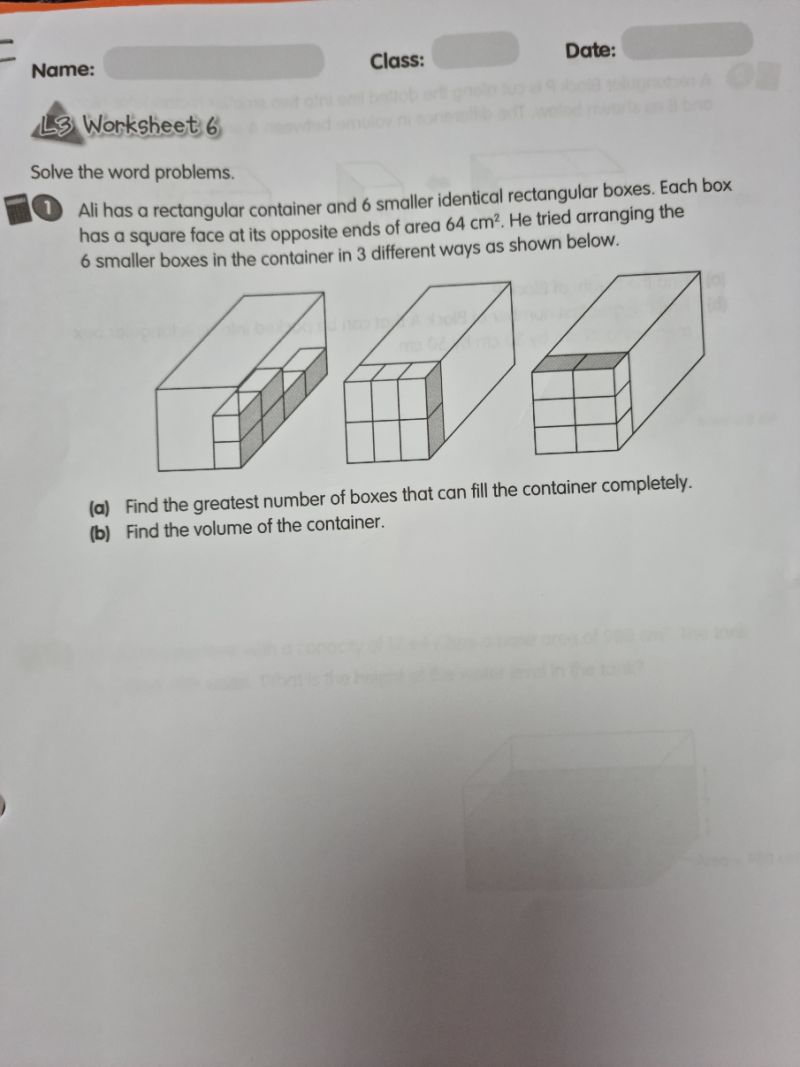# QuestionA bit confusing pls help. Thank you.

Let’s name the 3 diagrams A, B and C

From B and C, we know that 3 short side = 2 long sides of the small boxes

Sqroot(64) = 8 , each short side = 8, 3 sides = 8 x 3 = 24

So the long side = 24/2 = 12

Hence we know the dimensions of the big box from A, B and C.

The surface facing us is 8 x 8 and the depth is 12x 4 = 48

From A, the boxes to fill = 3 x 3 x 4 = 36

From B, the boxes to fill = 3 x 2 x 6 =36

From C, the boxes to fill = 2 x 3 x 6 = 36

(a) Greatest number of boxes to fill the container = 36

(b) Volume of the container = 8 x 8 x 48 = 3072 cm3

0 Replies 1 Like